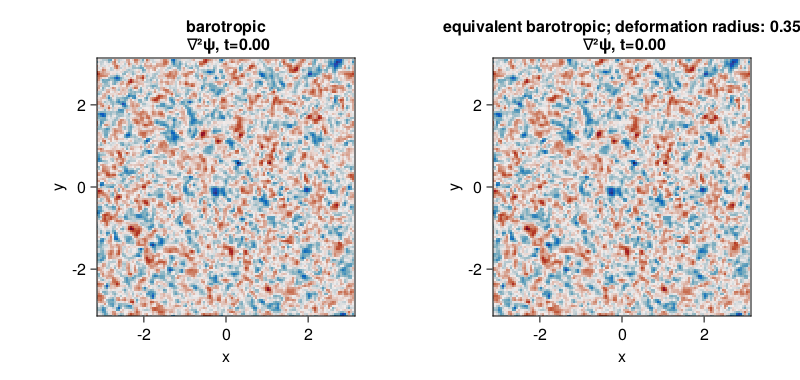# SingleLayerQG decaying 2D turbulence with and without finite Rossby radius of deformation

This example can be viewed as a Jupyter notebook via.

We use here the SingleLayerQG module to simulate decaying two-dimensional turbulence and investigate how does a finite Rossby radius of deformation affects its evolution.

## Install dependencies

First let's make sure we have all required packages installed.

using Pkg
pkg"add GeophysicalFlows, Printf, Random, CairoMakie"

## Let's begin

Let's load GeophysicalFlows.jl and some other packages we need.

using GeophysicalFlows, Printf, Random, CairoMakie

using GeophysicalFlows: peakedisotropicspectrum
using LinearAlgebra: ldiv!
using Random: seed!

## Choosing a device: CPU or GPU

dev = CPU()     # Device (CPU/GPU)

## Numerical, domain, and simulation parameters

First, we pick some numerical and physical parameters for our model.

n, L  = 128, 2π             # grid resolution and domain length

# Then we pick the time-stepper parameters
dt = 1e-2  # timestep
nsteps = 4000  # total number of steps
nsubs = 20    # number of steps between each plot

## Problem setup

We initialize two problems by providing a set of keyword arguments to the Problem constructor. The two problems are otherwise the same, except one has an infinite deformation radius, prob_bqg, and the other has finite deformation radius, prob_eqbqg.

For both problems we use stepper = "FilteredRK4". Filtered timesteppers apply a wavenumber-filter at every time-step that removes enstrophy at high wavenumbers and, thereby, stabilize the problem, despite that we use the default viscosity coefficient ν=0. Thus, we choose not to do any dealiasing by providing aliased_fraction=0.

stepper="FilteredRK4"

prob_bqg = SingleLayerQG.Problem(dev; nx=n, Lx=L, dt, stepper, aliased_fraction=0)
prob_eqbqg = SingleLayerQG.Problem(dev; nx=n, Lx=L, deformation_radius, dt, stepper, aliased_fraction=0)

## Setting initial conditions

For initial condition we construct a relative vorticity with energy most energy around total wavenumber $k_0$.

seed!(1234)
k₀, E₀ = 6, 0.5
∇²ψ₀ = peakedisotropicspectrum(prob_bqg.grid, k₀, E₀, mask=prob_bqg.timestepper.filter)

SingleLayerQG allows us to set up the initial $q$ for each problem via set_q!() function. To initialize both prob_bqg and prob_eqbqg with the same flow, we first use function SingleLayerQG.streamfunctionfrompv! to get the streamfunction that corresponds to the relative vorticity we computed above. This works in the purely barotropic problem, prob_bqg since in that case the QGPV is simply the relative vorticity.

∇²ψ₀h = rfft(∇²ψ₀)
ψ₀h = @. 0 * ∇²ψ₀h
SingleLayerQG.streamfunctionfrompv!(ψ₀h, ∇²ψ₀h, prob_bqg.params, prob_bqg.grid)

and then use the streamfunction to compute the corresponding $q_0$ for each problem,

q₀_bqg   = irfft(-prob_bqg.grid.Krsq .* ψ₀h, prob_bqg.grid.nx)
q₀_eqbqg = irfft(-(prob_eqbqg.grid.Krsq .+ 1/prob_eqbqg.params.deformation_radius^2) .* ψ₀h, prob_bqg.grid.nx)

Now we can initialize our problems with the same flow.

SingleLayerQG.set_q!(prob_bqg, q₀_bqg)
SingleLayerQG.set_q!(prob_eqbqg, q₀_eqbqg)

Let's plot the initial vorticity field for each problem. Note that when plotting, we decorate the variable to be plotted with Array() to make sure it is brought back on the CPU when vars live on the GPU.

function relativevorticity(prob)
vars, grid = prob.vars, prob.grid

ldiv!(vars.q, grid.rfftplan, - grid.Krsq .* vars.ψh)

return vars.q
end

x,  y  = prob_bqg.grid.x,  prob_bqg.grid.y
Lx, Ly = prob_bqg.grid.Lx, prob_bqg.grid.Ly

fig = Figure(resolution=(800, 380))

axis_kwargs = (xlabel = "x",
ylabel = "y",
aspect = 1,
limits = ((-Lx/2, Lx/2), (-Ly/2, Ly/2)))

t_bqg = Observable(prob_bqg.clock.t)
t_eqbqg = Observable(prob_eqbqg.clock.t)

title_bqg = @lift "barotropic\n ∇²ψ, t=" * @sprintf("%.2f", $t_bqg) title_eqbqg = @lift "equivalent barotropic; deformation radius: " * @sprintf("%.2f", prob_eqbqg.params.deformation_radius) * "\n ∇²ψ, t=" * @sprintf("%.2f",$t_eqbqg)

ax1 = Axis(fig[1, 1]; title = title_bqg, axis_kwargs...)
ax2 = Axis(fig[1, 2]; title = title_eqbqg, axis_kwargs...)

ζ_bqg = Observable(Array(relativevorticity(prob_bqg)))
ζ_eqbqg = Observable(Array(relativevorticity(prob_eqbqg)))

heatmap!(ax1, x, y, ζ_bqg;
colormap = :balance, colorrange = (-40, 40))

heatmap!(ax2, x, y, ζ_eqbqg;
colormap = :balance, colorrange = (-40, 40))

fig## Time-stepping the Problem forward

Now we time-step both problems forward and animate the relative vorticity in each case.

startwalltime = time()

cfl(prob) = prob.clock.dt * maximum([maximum(prob.vars.u) / prob.grid.dx, maximum(prob.vars.v) / prob.grid.dy])

record(fig, "singlelayerqg_barotropic_equivalentbarotropic.mp4", 0:Int(nsteps/nsubs), framerate = 18) do j
if j % (1000 / nsubs) == 0
log_bqg = @sprintf("barotropic; step: %04d, t: %d, cfl: %.2f, walltime: %.2f min",
prob_bqg.clock.step, prob_bqg.clock.t, cfl(prob_bqg), (time()-startwalltime)/60)
println(log_bqg)

log_eqbqg = @sprintf("equivalent barotropic; step: %04d, t: %d, cfl: %.2f, walltime: %.2f min",
prob_eqbqg.clock.step, prob_eqbqg.clock.t, cfl(prob_eqbqg), (time()-startwalltime)/60)
println(log_eqbqg)
end

stepforward!(prob_bqg, nsubs)
SingleLayerQG.updatevars!(prob_bqg)

stepforward!(prob_eqbqg, nsubs)
SingleLayerQG.updatevars!(prob_eqbqg)

t_bqg[] = prob_bqg.clock.t
t_eqbqg[] = prob_eqbqg.clock.t
ζ_bqg[] = relativevorticity(prob_bqg)
ζ_eqbqg[] = relativevorticity(prob_eqbqg)
end
barotropic; step: 0000, t: 0, cfl: 0.48, walltime: 0.00 min
equivalent barotropic; step: 0000, t: 0, cfl: 0.48, walltime: 0.00 min
barotropic; step: 1000, t: 10, cfl: 0.65, walltime: 0.11 min
equivalent barotropic; step: 1000, t: 10, cfl: 0.46, walltime: 0.11 min
barotropic; step: 2000, t: 20, cfl: 0.52, walltime: 0.21 min
equivalent barotropic; step: 2000, t: 20, cfl: 0.35, walltime: 0.21 min
barotropic; step: 3000, t: 30, cfl: 0.48, walltime: 0.31 min
equivalent barotropic; step: 3000, t: 30, cfl: 0.32, walltime: 0.31 min
barotropic; step: 4000, t: 40, cfl: 0.49, walltime: 0.42 min
equivalent barotropic; step: 4000, t: 40, cfl: 0.34, walltime: 0.42 min